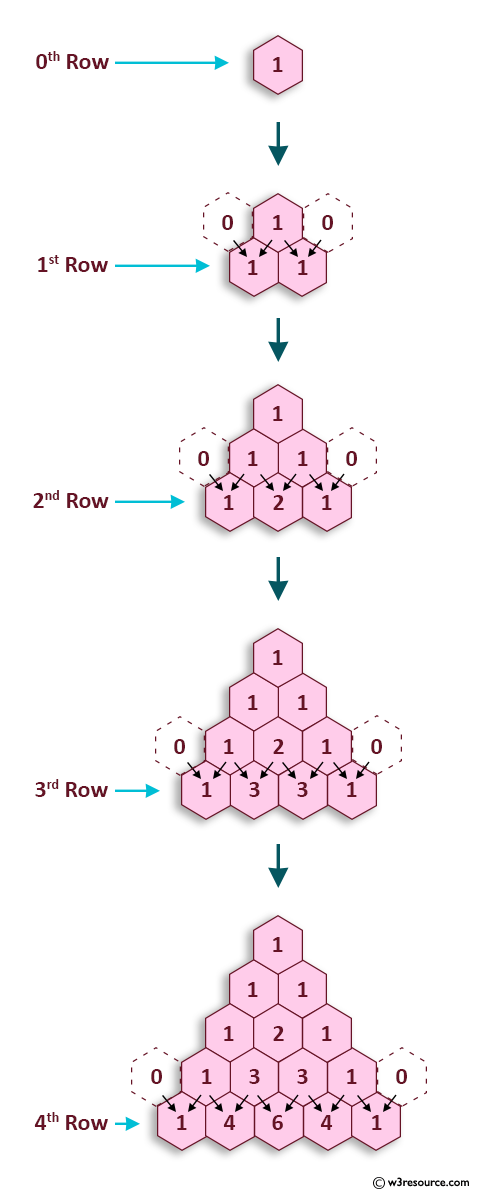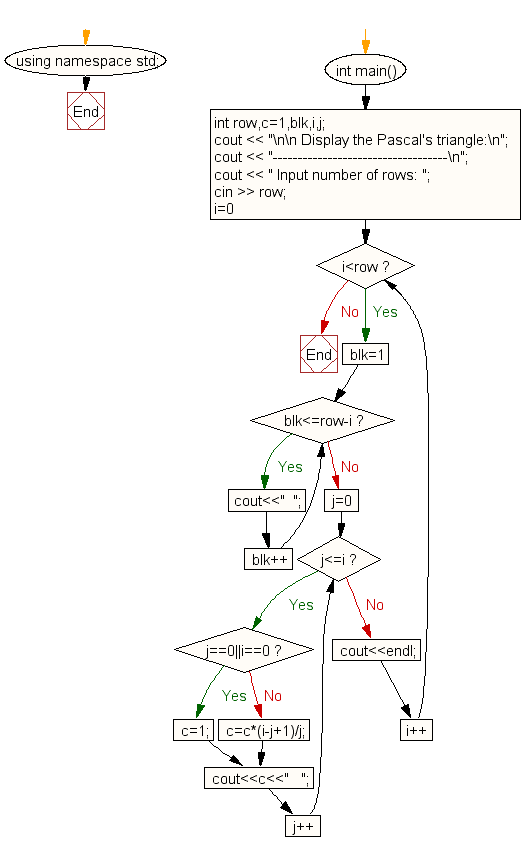﻿ C++ Exercises: Display Pascal's triangle like pyramid - w3resource

# C++ Exercises: Display Pascal's triangle like pyramid

## C++ For Loop: Exercise-45 with Solution

Write a C++ program to display Pascal's triangle like a pyramid.

Construction of Pascal's Triangle:

As shown in Pascal's triangle, each element is equal to the sum of the two numbers immediately above it.Sample Solution:

C++ Code :

``````#include <iostream>
using namespace std;

int main()
{
int row,c=1,blk,i,j;
cout << "\n\n Display the Pascal's triangle:\n";
cout << "-----------------------------------\n";
cout << " Input number of rows: ";
cin >> row;
for(i=0;i<row;i++)
{
for(blk=1;blk<=row-i;blk++)
cout<<"  ";
for(j=0;j<=i;j++)
{
if (j==0||i==0)
c=1;
else
c=c*(i-j+1)/j;
cout<<c<<"   ";
}
cout<<endl;
}
}
``````

Sample Output:

``` Display the Pascal's triangle:
-----------------------------------
Input number of rows: 5
1
1   1
1   2   1
1   3   3   1
1   4   6   4   1
```

Flowchart:C++ Code Editor: